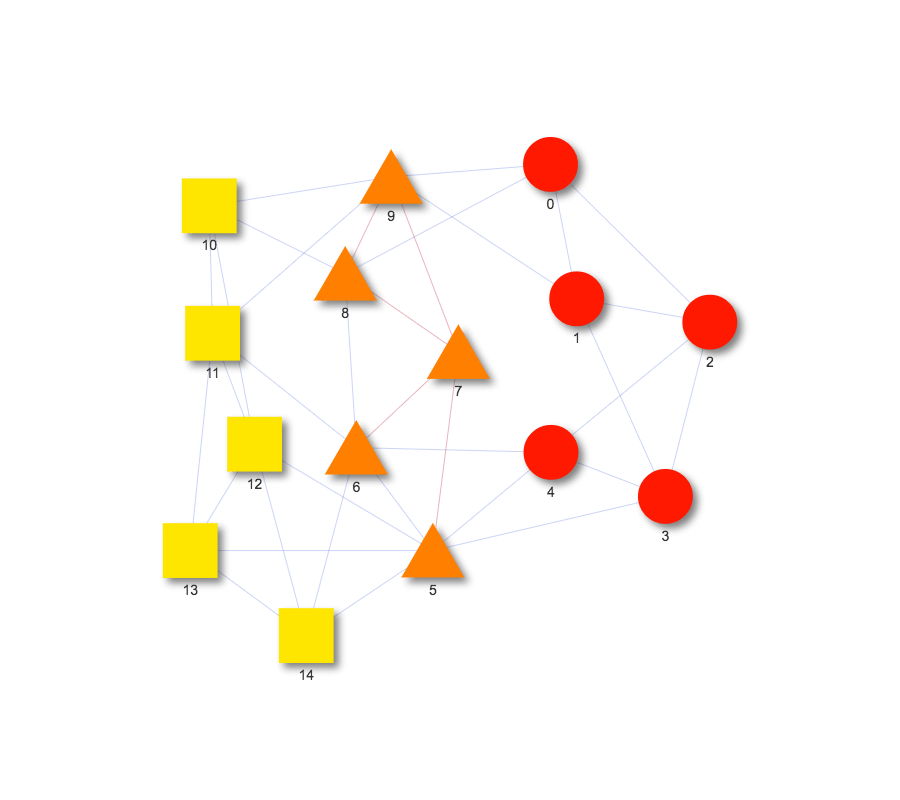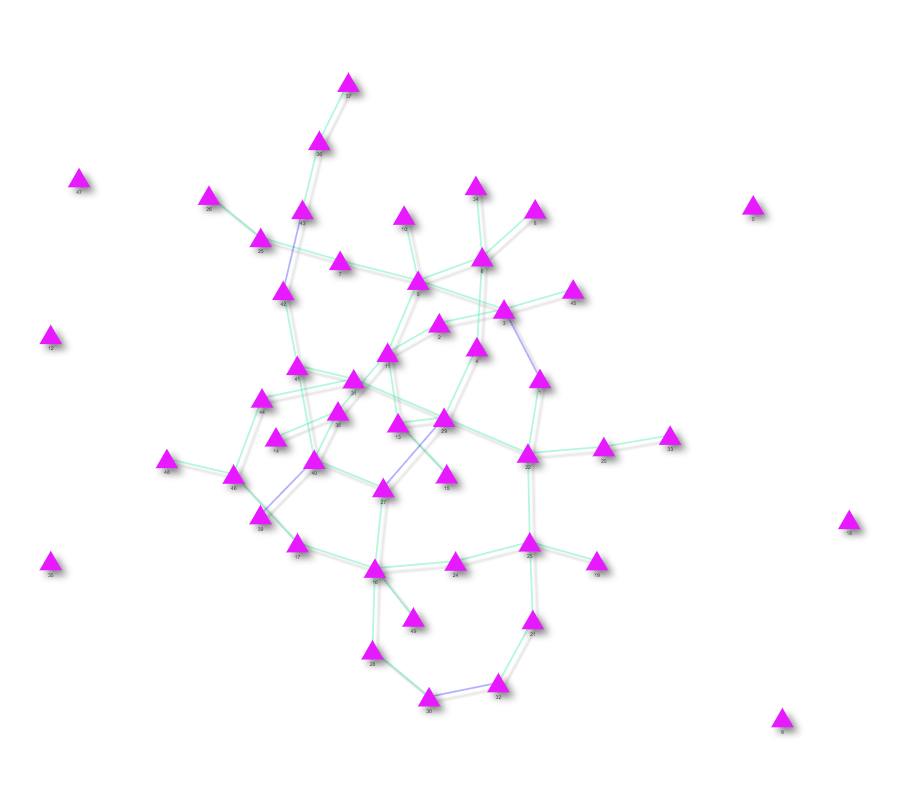## Visualizing the similarity of two networks

Julia Len (jlen at ucsd.edu)

### Introduction

When working with networks, it is often useful to consider how similar two networks are.  There are a number of ways of quantifying network similarity however.  One could simply consider the number of nodes two networks have in common.  However, this would miss any structural similarity, or lack thereof, between the edges.  For example, it is possible for two networks to have completely identical node sets, but have completely disjoint edge sets.  Note however that in order for two networks to share edges, they must share nodes as well (since edges are defined by the nodes they connect).

In this post, we will introduce a network overlap visualization function (draw_graph_union) in the visJS2jupyter package, and explore a few possible scenarios.

### Installation

To install visJS2jupyter, run

`    pip install visJS2jupyter`

in your terminal. To import the visualizations module, use the statement

`    import visJS2jupyter.visualizations as visualizations`

in your jupyter notebook. The source code is also available on github here.

### Simple example with default parameters

We will now go through a simple example using two 10-node networks whose intersection is exactly 5 nodes. We create two small, random networks using the networkx function ‘connected_watts_strogatz_graph’. Each network will have 10 nodes, with each node initially connected to its 5 nearest neighbors. These connections are then randomly rewired with probability 0.1.

`    G1 = nx.connected_watts_strogatz_graph(10,5,.1)`

`    G2 = nx.connected_watts_strogatz_graph(10,5,.1)`

This produces two networks who both have nodes labelled from 0 to 9. Their intersection is then all the nodes for each graph. This is an unexciting case, so let’s relabel some nodes, so that they share only 5 nodes in common. We can do this by relabelling the nodes 0 to 9 of the second graph, G2, to 5 to 14 using the networkx function ‘relabel_nodes’. The code for this is shown below:

`    old_nodes = range(5)`

`    new_nodes = range(10,15)`

`    new_node_labels = dict(zip(old_nodes,new_nodes))`

`    G2 = nx.relabel_nodes(G2,new_node_labels)`

Now nodes 0 to 4 belong to only G1, nodes 5 to 9 belong to G1 and G2, and nodes 10 to 14 belong to only G2. Let’s see what this looks like by using draw_graph_union:

`    visualizations.draw_graph_union(G1,G2)`And that’s it! We get an interactive graph fairly quickly and easily. Notice that the nodes are color-coded and shaped based on which network they belong to. For instance, nodes in the intersection of G1 and G2 are orange and triangular shapes, while nodes which only belong to G1 are red circles, and nodes which only belong to G2 are yellow squares. Also notice that edges found in both G1 and G2 are colored red while all other edges are colored blue.

You can take a look at the sample notebook here.  Notice that hovering over a node pops up a tooltip with information about the node’s name and graph membership.

From the previous example, we saw that draw_graph_union not only depicts the intersection of nodes, but it also visualizes the intersection of edges as well. Let’s take a look at how this works with two networks having identical nodes but but only a few overlapping edges.

### Identical nodes, some overlapping edges

We’ll again be using the connected_watts_strogatz_graph to create our two networks, but this time both networks will contain 50 nodes:

`    G1 = nx.connected_watts_strogatz_graph(50,5,.1)`

`    G2 = nx.connected_watts_strogatz_graph(50,5,.1)`

This produces two networks with identical nodes and randomly intersecting edges. We want the sets of edges to only intersect over 5 nodes. Python’s built-in set object can help with this. We can get the edges for G1 and G2, convert the lists of edges to sets of edges, and then find their intersection using &. We can then subtract out the intersecting edges from each set of edges.

`    edges_1 = set(G1.edges())`

`    edges_2 = set(G2.edges())`

`    intersecting_edges = edges_1 & edges_2`

`    edges_1_disjoint = edges_1 - intersecting_edges`

`    edges_2_disjoint = edges_2 - interesecting_edges`

This produces two disjoint sets of edges. We now want to add back in 5 edges from the intersection into each disjoint set.

`    for i in range(0,5):`

`        new_edge = intersecting_edges.pop()`

`        edges_1_disjoint.add(new_edge)`

`        edges_2_disjoint.add(new_edge)`

We can then remove the current edges from G1 and add back in the desired edges. We do the same for G2.

`    G1.remove_edges_from(edges_1)`

`    G1.add_edges_from(list(edges_1_disjoint))`

Let’s now draw the two graphs using draw_graph_union, but this time let’s customize the graph a bit. The function sets the default color of the nodes to matplotlib’s colormap autumn and the default color of the edges to matplotlib’s colormap coolwarm. However, matplotlib has many wonderful colormaps available that we can choose from (click here for more details). To set the colormap of the nodes and edges, use the arguments node_cmap and edge_cmap. If you decide to change the colormap, make sure to import the matplotlib package:

`    import matplotlib as mpl`

We can add other customizations as well, such as setting edge width and edge shadows. The function allows for any argument available in visJS_module in the visJS2jupyter package. This allows many potential customizing features for the function. Now, let’s see what this looks like overall:

`    visualizations.draw_graph_union(G1,G2,`

`        node_cmap=mpl.cm.cool,`

`        edge_cmap=mpl.cm.winter_r,`

`        edge_width=5,`

`        edge_shadow_enabled=True,`

`        edge_shadow_size=2)`As you can see in the graph above, there is now only one set of nodes, all of which are triangle shaped because all the nodes overlap. The edges are mostly colored in green except for 5 edges in blue: the edges in the intersection. Notice that the edges and nodes are colored differently from before and the edges now have added shadows. You can take a look at the interactive notebook with this example here.

### One network contains the other network

So far, we’ve seen graphs where there is a small intersection of the nodes and the node sets are equal. What happens if the set of nodes for one graph is a subset of the nodes for the other graph? We’ll take a look at this case now.

We again create two networks using connected_watts_strogatz. Graph 1 will have 50 nodes and graph 2 will have 20 so that all of the second graph’s nodes intersect with graph 1. We will then call draw_graph_union on these two graphs. This time, we use some more features of the function. We can set the name of the nodes for graph 1 and graph 2. Notice that previously when we hovered over a node, the tooltip showed something like “graph 1 + graph 2”. Using the arguments node_name_1 and node_name_2, we can customize what is shown in the tooltip. Pretty cool!

If you’ve played around with some of the example notebooks, you’ve probably noticed that the nodes move around when dragged as if they have a gravitational field. This is the physics_enabled feature. It is set by default for graphs of less than 100 nodes, while it is turned off for any larger graphs. One nice feature is that you can override this by setting the physics_enabled argument to true or false. Let’s turn off this setting for this example.

`    visualizations.draw_graph_union(G1,G2,edge_width=5`

`        node_name_1=”superset”,`

`        node_name_2=”subset”,`

`        physics_enabled=False)`In just a couple of lines of code, we have produced an interactive network! Notice that when we hover over a node, it has the name that we set, just like we wanted. You can also see that dragging a node around makes it stay stuck in place, so the physics_enabled setting has been turned off. The example notebook can be found here.

Overall, draw_graph_union provides a quick and easy way to create customizable and interactive visualizations for network similarities, enabling visual assessment of what two networks share and what they don’t.

« Return